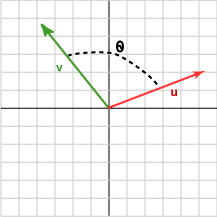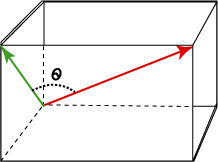180° (or π radians)

# The Dot ProductFor geometrical vectors in two or three dimensional space, the dot product of two vectors u and v is:

 u · v = |u| |v| cos θ

The dot product is indicated by the dot between the two vectors. Unfortunately, many browsers do not show the dot very clearly. You may see a very tiny dot or a small black bar. θ is the angle between the two vectors.

Don't write two vectors next to each other like this: uv when you want the dot product. Always put a dot between them: u · v .

In 2D the two vectors lie in a plane (of course) and the angle between them is easy to visualize.

In 3D, two vectors also lie in a plane embedded within the 3D space except when the two vectors point in the same direction.

When two vectors point in the same direction, the angle between them is zero and so:

u · v = |u||v| cos 0 = |u||v| 1 = |u||v|

Since -1 <= cos θ <= +1 the value of the dot product is between -|u||v| and +|u||v| depending on the angle between the vectors.

When two vectors point in opposite directions, the angle between them is 180° and the dot product is -|u||v| .

### QUESTION 3:

If u · v = |u| |v| cos θ , what is v · u ?